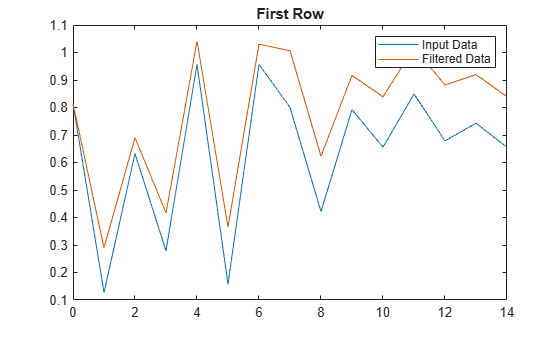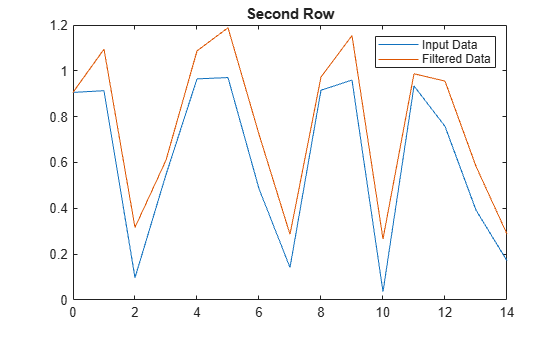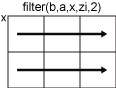filter

1-D digital filter

Description

example

y = filter(b,a,x) filters the input data x using a rational transfer function defined by the numerator and denominator coefficients b and a.

If a(1) is not equal to 1, then filter normalizes the filter coefficients by a(1). Therefore, a(1) must be nonzero.

• If x is a vector, then filter returns the filtered data as a vector of the same size as x.

• If x is a matrix, then filter acts along the first dimension and returns the filtered data for each column.

• If x is a multidimensional array, then filter acts along the first array dimension whose size does not equal 1.

example

y = filter(b,a,x,zi) uses initial conditions zi for the filter delays. The length of zi must equal max(length(a),length(b))-1.

example

y = filter(b,a,x,zi,dim) acts along dimension dim. For example, if x is a matrix, then filter(b,a,x,zi,2) returns the filtered data for each row.

example

[y,zf] = filter(___) also returns the final conditions zf of the filter delays, using any of the previous syntaxes.

Examples

collapse all

A moving-average filter is a common method used for smoothing noisy data. This example uses the filter function to compute averages along a vector of data.

Create a 1-by-100 row vector of sinusoidal data that is corrupted by random noise.

t = linspace(-pi,pi,100);
rng default  %initialize random number generator
x = sin(t) + 0.25*rand(size(t));

A moving-average filter slides a window of length $windowSize$ along the data, computing averages of the data contained in each window. The following difference equation defines a moving-average filter of a vector $x$:

$y\left(n\right)=\frac{1}{windowSize}\left(x\left(n\right)+x\left(n-1\right)+...+x\left(n-\left(windowSize-1\right)\right)\right).$

For a window size of 5, compute the numerator and denominator coefficients for the rational transfer function.

windowSize = 5;
b = (1/windowSize)*ones(1,windowSize);
a = 1;

Find the moving average of the data and plot it against the original data.

y = filter(b,a,x);

plot(t,x)
hold on
plot(t,y)
legend('Input Data','Filtered Data')This example filters a matrix of data with the following rational transfer function.

$H\left(z\right)=\frac{b\left(1\right)}{a\left(1\right)+a\left(2\right){z}^{-1}}=\frac{1}{1-0.2{z}^{-1}}$

Create a 2-by-15 matrix of random input data.

rng default  %initialize random number generator
x = rand(2,15);

Define the numerator and denominator coefficients for the rational transfer function.

b = 1;
a = [1 -0.2];

Apply the transfer function along the second dimension of x and return the 1-D digital filter of each row. Plot the first row of original data against the filtered data.

y = filter(b,a,x,[],2);

t = 0:length(x)-1;  %index vector

plot(t,x(1,:))
hold on
plot(t,y(1,:))
legend('Input Data','Filtered Data')
title('First Row')Plot the second row of input data against the filtered data.

figure
plot(t,x(2,:))
hold on
plot(t,y(2,:))
legend('Input Data','Filtered Data')
title('Second Row')Use initial and final conditions for filter delays to filter data in sections, especially if memory limitations are a consideration.

Generate a large random data sequence and split it into two segments, x1 and x2.

x = randn(10000,1);

x1 = x(1:5000);
x2 = x(5001:end);

The whole sequence, x, is the vertical concatenation of x1 and x2.

Define the numerator and denominator coefficients for the rational transfer function,

$H\left(z\right)=\frac{b\left(1\right)+b\left(2\right){z}^{-1}}{a\left(1\right)+a\left(2\right){z}^{-1}}=\frac{2+3{z}^{-1}}{1+0.2{z}^{-1}}.$

b = [2,3];
a = [1,0.2];

Filter the subsequences x1 and x2 one at a time. Output the final conditions from filtering x1 to store the internal status of the filter at the end of the first segment.

[y1,zf] = filter(b,a,x1);

Use the final conditions from filtering x1 as initial conditions to filter the second segment, x2.

y2 = filter(b,a,x2,zf);

y1 is the filtered data from x1, and y2 is the filtered data from x2. The entire filtered sequence is the vertical concatenation of y1 and y2.

Filter the entire sequence simultaneously for comparison.

y = filter(b,a,x);

isequal(y,[y1;y2])
ans = logical
1

Input Arguments

collapse all

Numerator coefficients of the rational transfer function, specified as a vector.

Data Types: double | single | int8 | int16 | int32 | int64 | uint8 | uint16 | uint32 | uint64 | logical
Complex Number Support: Yes

Denominator coefficients of the rational transfer function, specified as a vector.

Data Types: double | single | int8 | int16 | int32 | int64 | uint8 | uint16 | uint32 | uint64 | logical
Complex Number Support: Yes

Input data, specified as a vector, matrix, or multidimensional array.

Data Types: double | single | int8 | int16 | int32 | int64 | uint8 | uint16 | uint32 | uint64 | logical
Complex Number Support: Yes

Initial conditions for filter delays, specified as a vector, matrix, or multidimensional array.

• If zi is a vector, then its length must be max(length(a),length(b))-1.

• If zi is a matrix or multidimensional array, then the size of the leading dimension must be max(length(a),length(b))-1. The size of each remaining dimension must match the size of the corresponding dimension of x. For example, consider using filter along the second dimension (dim = 2) of a 3-by-4-by-5 array x. The array zi must have size [max(length(a),length(b))-1]-by-3-by-5.

The default value, specified by [], initializes all filter delays to zero.

Data Types: double | single | int8 | int16 | int32 | int64 | uint8 | uint16 | uint32 | uint64 | logical
Complex Number Support: Yes

Dimension to operate along, specified as a positive integer scalar. If no value is specified, then the default is the first array dimension whose size does not equal 1.

Consider a two-dimensional input array, x.

• If dim = 1, then filter(b,a,x,zi,1)works along the rows of x and returns the filter applied to each column.• If dim = 2, then filter(b,a,x,zi,2) works along the columns of x and returns the filter applied to each row.If dim is greater than ndims(x), then filter returns x.

Data Types: double | single | int8 | int16 | int32 | int64 | uint8 | uint16 | uint32 | uint64 | logical

Output Arguments

collapse all

Filtered data, returned as a vector, matrix, or multidimensional array of the same size as the input data, x.

If x is of type single, then filter natively computes in single precision, and y is also of type single. Otherwise, y is returned as type double.

Data Types: double | single

Final conditions for filter delays, returned as a vector, matrix, or multidimensional array.

• If x is a vector, then zf is a column vector of length max(length(a),length(b))-1.

• If x is a matrix or multidimensional array, then zf is an array of column vectors of length max(length(a),length(b))-1, such that the number of columns in zf is equivalent to the number of columns in x. For example, consider using filter along the second dimension (dim = 2) of a 3-by-4-by-5 array x. The array zf has size [max(length(a),length(b))-1]-by-3-by-5.

Data Types: double | single

collapse all

Rational Transfer Function

The input-output description of the filter operation on a vector in the Z-transform domain is a rational transfer function. A rational transfer function is of the form

$Y\left(z\right)=\frac{b\left(1\right)+b\left(2\right){z}^{-1}+...+b\left({n}_{b}+1\right){z}^{-{n}_{b}}}{1+a\left(2\right){z}^{-1}+...+a\left({n}_{a}+1\right){z}^{-{n}_{a}}}X\left(z\right),$

which handles both FIR and IIR filters . na is the feedback filter order, and nb is the feedforward filter order. Due to normalization, assume a(1) = 1.

You also can express the rational transfer function as the difference equation

$\begin{array}{c}a\left(1\right)y\left(n\right)=b\left(1\right)x\left(n\right)+b\left(2\right)x\left(n-1\right)+...+b\left({n}_{b}+1\right)x\left(n-{n}_{b}\right)\\ -a\left(2\right)y\left(n-1\right)-...-a\left({n}_{a}+1\right)y\left(n-{n}_{a}\right).\end{array}$

Furthermore, you can represent the rational transfer function using its direct-form II transposed implementation, as in the following diagram. Here, na = nb.The operation of filter at sample m is given by the time-domain difference equations

Tips

• To use the filter function with the b coefficients from an FIR filter, use y = filter(b,1,x).

• If you have Signal Processing Toolbox™, use y = filter(d,x) to filter an input signal x with a digitalFilter (Signal Processing Toolbox) object d. To generate d based on frequency-response specifications, use designfilt (Signal Processing Toolbox).

• See Digital Filtering (Signal Processing Toolbox) for more on filtering functions.

 Oppenheim, Alan V., Ronald W. Schafer, and John R. Buck. Discrete-Time Signal Processing. Upper Saddle River, NJ: Prentice-Hall, 1999.